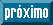Articulo

•Citado por SciELO
•Accesos

•Similares en SciELO

versión impresa ISSN 1409-2433

Resumen

SIMANCAS-GARCIA, José L.  y  GEORGE-GONZALEZ, Kemel. Discrete sampling theorem to Shannon’s sampling theorem using the hyperreal numbers ∗R. Rev. Mat [online]. 2021, vol.28, n.2, pp.163-182. ISSN 1409-2433.  http://dx.doi.org/10.15517/rmta.v28i2.43356.

Shannon’s sampling theorem is one of the most important results of modern signal theory. It describes the reconstruction of any band-limited signal from a finite number of its samples. On the other hand, although less well known, there is the discrete sampling theorem, proved by Cooley while he was working on the development of an algorithm to speed up the calculations of the discrete Fourier transform. Cooley showed that a sampled signal can be resampled by selecting a smaller number of samples, which reduces computational cost. Then it is possible to reconstruct the original sampled signal using a reverse process. In principle, the two theorems are not related. However, in this paper we will show that in the context of Non-Standard Mathematical Analysis (NSA) and Hyperreal Numerical System ∗R, the two theorems are equivalent. The difference between them becomes a matter of scale. With the scale changes that the hyperreal number system allows, the discrete variables and functions become continuous, and Shannon’s sampling theorem emerges from the discrete sampling theorem.

Palabras clave : Sampling theorem; subsampling; hyperreal number system; infinitesimal calculus model..

· resumen en Español     · texto en Inglés     · Inglés (pdf )